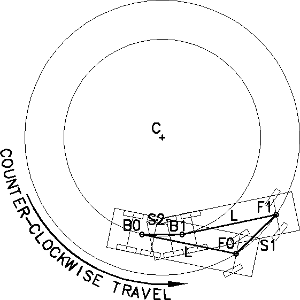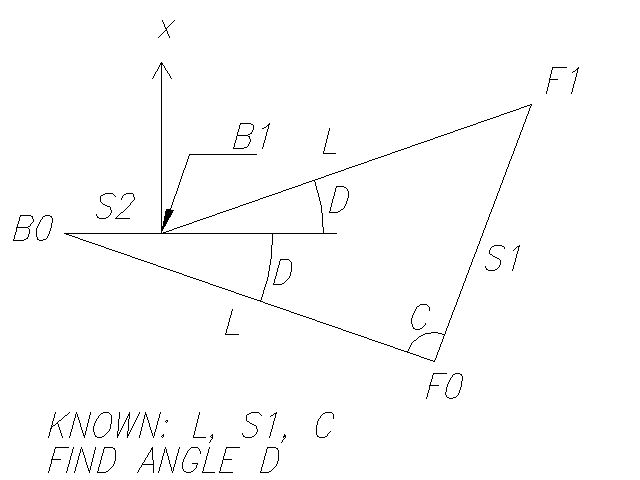# TURN.LSP Theory

>> Drop your fears at the door; love is spoken here. Enjoy the free libre HawsEDC AutoCAD tools too. <<

 HawsEDC Engineering, Drafting, and Computing

## TURN.LSP TURNING MODEL THEORY

THEORY

For each computation step, a vehicle wheel (or axle set) pair (front and back) is assumed to be traveling in a circle as though the steering wheel or articulating hinge were locked. The front and back wheels (axle sets) are circumscribing two concentric circles, with the line between back and front wheel always tangent to the inner circle being made by the back wheel, as shown:The initial locations of the back and front wheels (axle sets) are known (B0 and F0), and the final location of the front wheel is known (F1).

Then the turned angle F0|C|F1=B0|C|B1=2*atan{sin(alpha)/[2*L/S1-cos(alpha)]}

where

S1=the distance from F0 to F1
L=the distance from B0 to F0, the wheelbase length
alpha=angle F1|F0|B0

Vector addition is the method for deriving this equation.The sum of the x components traveling around the shape from B0 to F0, F1, B1, and back to B0 should be zero.
Then -L*sin(D)+S1*sin(pi-(C+D))-L*sin(D)+0=0
and gathering terms, S1*sin (pi-(C+D))-2*L*sin(D)=0, or S1*sin (pi-(C+D))=2*L*sin(D).

Since sin(pi-t)=sin(t) (http://aleph0.clarku.edu/~djoyce/java/trig/identities.html),
S1*sin(C+D)=2*L*sin(D)

Since sin(s+t)=sin(s)*cos(t)+cos(s)*sin(t) (http://aleph0.clarku.edu/~djoyce/java/trig/identities.html),
S1*sin(C)*cos(D)+S1*cos(C)*sin(D)=2*L*sin(D).

Dividing by S1*sin(D) and replacing cos(D)/sin(D) with cot(D),
sin(C)*cot(D)+cos(C)=2*L/S1

Solving for D,
sin(C)*cot(D)=2*L/S1-cos(C),
cot(D)=2*L/(S1*sin(C))-cot(C),
cot(D)=[2*L/(S1)-cos(C)]/sin(C),
tan(D)=sin(C)/[2*L/(S1)-cos(C)],
and D=atan{sin(C)/[2*L/(S1)-cos(C)]}